# RW-40-5 Single-Ended 3K / 5K ohms 40W type Audio Output Transformer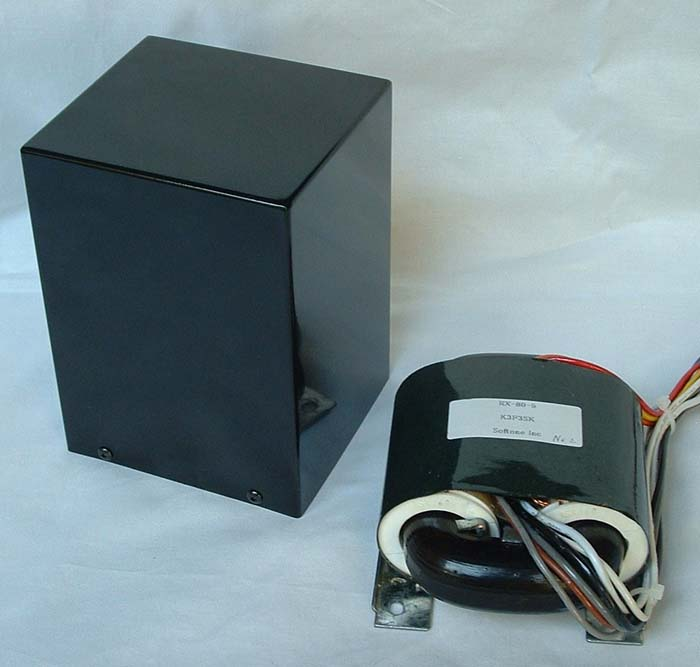The right on the photo is the R core output transformer in bare condition. The product is enclosed in the square case on the left.

RW-40-5 is R core audio output transformer for single ended type amplifier. Excellent magnetic properties of R core as well as precisely wound construction of the coil by the computerized manufacturing system achieve the wide bandwidth, low distortion, and low magnetic loss of the transformer.

### Outline

• Power handling capacity 40W, primary impedance 3,000 ohms or 5,000ohms, equipped with ultra-linear taps and cathode feedback winding, the best match for single ended tube amplifier using 300B,6CA7/EL34,KT88,6550,EL156, etc.
• High power, the low distortion , and the high dumping factor are obtained by the cathode feedback circuit.
• The cathode feedback winding is suitable for the amplifier of high internal resistance transmitter tube such as 808,811,100TH.
• Push-pull connection is also available. You can use this transformer as constant inductance type push-pull output transformer. With RW-40-5, you can build a maintenance-free push-pull amplifier.
• The combination of high performance core and generous turns of coil winding assures high primary inductance presenting good sound quality such as solidness of mid and low range.
• Precisely wound coil has no peculiar peaks and dips in attenuation characteristic and excellent response at high frequency, so enabling stable NFB operation and transparent sound quality.
• Perfect balance coil design realizes the low distortion factor even with non-NFB amplifier.
• Enclosed in a handy and beautiful square case. The same mounting dimensions as those of Tango XE60, FC-30.
• The case color is a black and silver.

### Specifications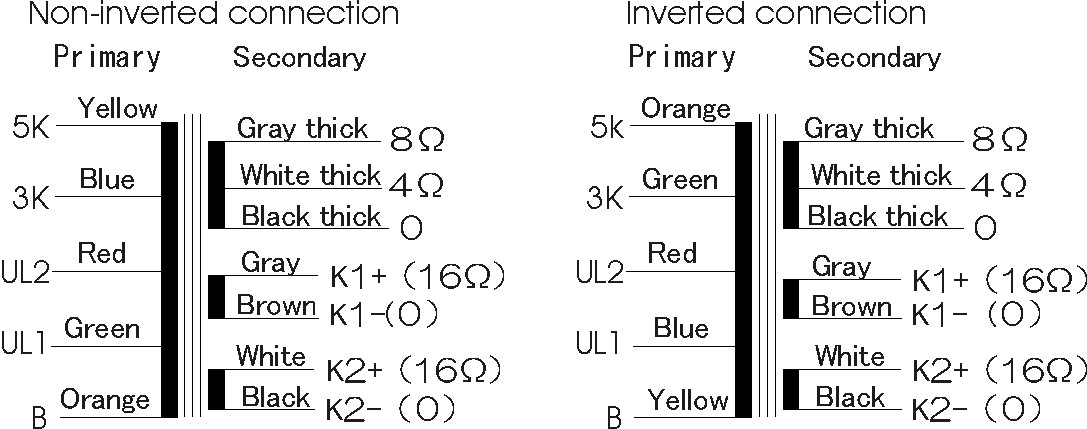Type Single-Ended Output capacity 40W at 35Hz Primary impedance 3,000 ohms, 5,000 ohms, with UL tap Secondary impedance 4 and 8 ohms 3rd. cathode feedback winding 16 ohms X 2 Frequency bandwidth 25Hz to 63Khz (-2dB), input=4V, signal source impedance=3,000 ohms 25Hz to 60Khz (-2dB), input=4V, signal source impedance=5,000 ohms Primary inductance (H) 12H(min.), 15H(max.) (3,000 ohms, DC current 80mA) 19H(min.), 23H(max.) (5,000 ohms, DC current 80mA) Primary permissible DC current 140mA(3,000 ohms), 120mA(5,000 ohms) Recommended primary DC current 110mA or less (3,000 ohms), 90mA or less (5,000 ohms) Power loss 0.23dB 8ohms DC resistance Primary B to 9.5K : 168ohms Secondary 0 to 8 ohms : 0.17ohms 3rd. 0 to 16 ohms : 4.18 ohms Dielectric withstanding voltage between primary, secondary and 3rd. . 2KV AC Maximum permissible voltage of primary B to 9.5K 1KV AC Core Type R160 160W core Shape Enclosed in square case, the same mounting dimensions as those of Tango XE60,FC-30 Connections Lead wires Overall dimensions and weight W: 110mm, D: 100mm, H: 150mm, Wt.: 3Kg W: 4.33inch, D: 3.94inch, H: 5.91inch, Wt.: 6.6lbs. Case color black, silver Price US\$220 (excluding tax and shipping)

### Selection of load impedance and phase

The table below describes the selection of load impedance and the relation of the phase between the primary and secondary and 3rd. You can select non-inverted connection between the primary and secondary and 3rd for two-stage amplifier and inverted connection for three-stage amplifier. When the load is selected to 5,000 ohms, two taps for UL are available; its feedback factor is 50% or 25%. The higher factor makes the plate resistance and distortion lower, however, the sensibility and output power will be reduced.

 Connected to Non-inverted 5,000 ohms Non-inverted 3,000 ohms Inverted 5,000 ohms Inverted 3,000 ohms Plate Yellow Blue Orange Green Screen grid (UL tap factor) Red(50%) Green (25%) Green (33%) Red (50%) Blue (25%) Blue (33%) Power source Orange Orange Yellow Yellow

### Cathode feedback winding

When using the cathode feedback winding, the connection of primary winding and cathode feedback winding is as follows.
There are two cathode feedback windings in RW-40-5. The cathode feedback ratio can be selected by two windings being used in parallel or in series.
The cathode feedback ratio decreases if it uses in parallel. The cathode feedback ratio increases if it uses in series.
In addition, a different wire connection adjusts to RW-40-5 by the Non-inverted or the Inverted. The table below shows the connection of each situation.

 Non-inverted Inverted cathode feedback winding parallel Low feedback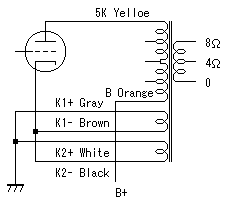Non-inverted Inverted cathode feedback winding series High feedback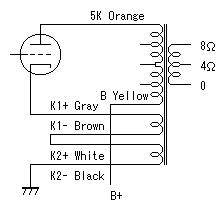Calculating the closed-loop gain and feedback ratio
It explains here as 5K Primary and in parallel cathode feedback winding.
The gain and ratio for 3K Primary and in series cathode feedback winding is described in the last table.

The load of the output tube is both the primary winding and cathode feedback winding in the cathode feedback circuit.
The load impedance of the output tube is not 5K ohms.
The load impedance is as follows.

Rl = ( SQRT(5000) + SQRT(16) ) **2 = 5582 ohms
SQRT    : Square route operation
**           : Factorial operation

The open-loop gain on the primary side before feedback
Aopen = gm * ( 5582 // Rp )
gm         : Transconductance of the output tube
Rp         : Plate resistance of the output tube
//            : Parallel value operation

The feedback factor β is a voltage ratio (a winding ratio) of load Rl and the cathode winding .
β = SQRT ( 16 / 5582 ) = 0.054

The closed loop gain on the primary side after feedback
Aclosed = Aopen / ( 1 + Aopen * β )

Feedback ratio = 20 * log( Aopen / Aclosed ) dB

Example
Value that uses EL34 for output tube
gm of EL34 = 11000uS
Rp of EL34 = 15000 ohms

The open-loop gain on the primary side before feed back
Aopen   = gm * ( 5582 // Rp )
= 11000 * 10**-6 ( 5582 // 15000 )
= 11000 * 10**-6 * 4068
= 11 * 4.068 = 44.75

The closed loop gain on the primary side after feedback
Aclosed = Aopen / ( 1 + Aopen * β )
= 44.75 / ( 1 + 44.75 * 0.054 )
= 44.75 / 3.42 = 13.08
The feedback ratio
Feedback ratio   = 20 * log( Aopen / Aclosed ) dB
= 20 * log( 44.75 / 13.08 ) = 10.68 dB

 Primary 5K parallel cathode feedback winding Primary 5K series cathode feedback winding Primary 3K parallel cathode feedback winding Primary 3K series cathode feedback winding Impedance of cathode feedback winding 16 64 16 64 load ImpedanceRl 5582 6195 3454 3940 Feedback factor β 0.054 0.101 0.068 0.127 Open-loop gain by EL34 44.75 48.22 30.8 34.32 Closed loop gain by EL34 13.08 8.21 9.97 6.4 Feedback ratioBy EL34 3.42 10.68dB 5.87 15.37dB 3.09 9.79dB 5.36 14.58dB

### Shape and Dimensions

• Transformer cover case facilitates mounting on the amplifier chassis and brings superior looking to the amplifier.
• The same mounting dimensions as those of Tango XE60,FC-30.
• The beautifully finished case is four-side welded construction, black or silver painted.
• Mounting and overall dimensions

### Characteristics

1. Frequency Characteristics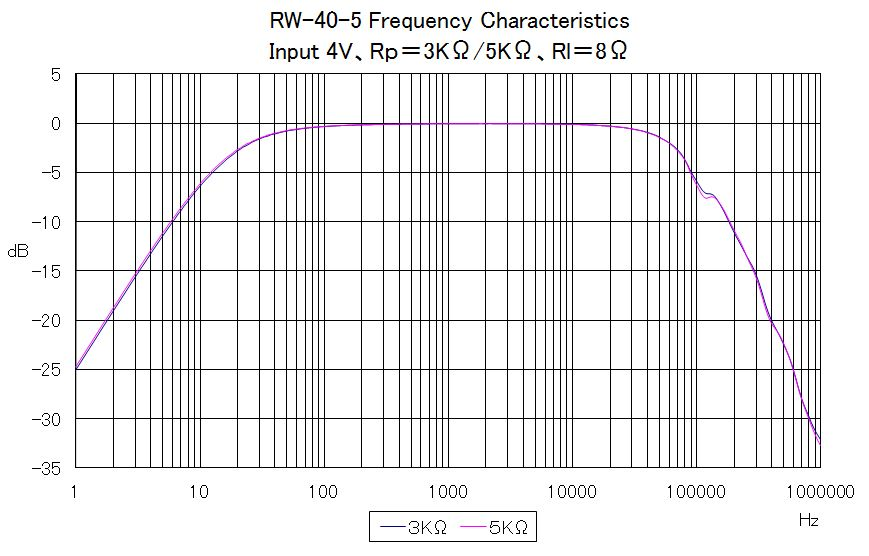2. Frequency Characteristic: with different signal source impedances

The frequency characteristic of the audio output transformer varies with the signal source impedance that drives the transformer.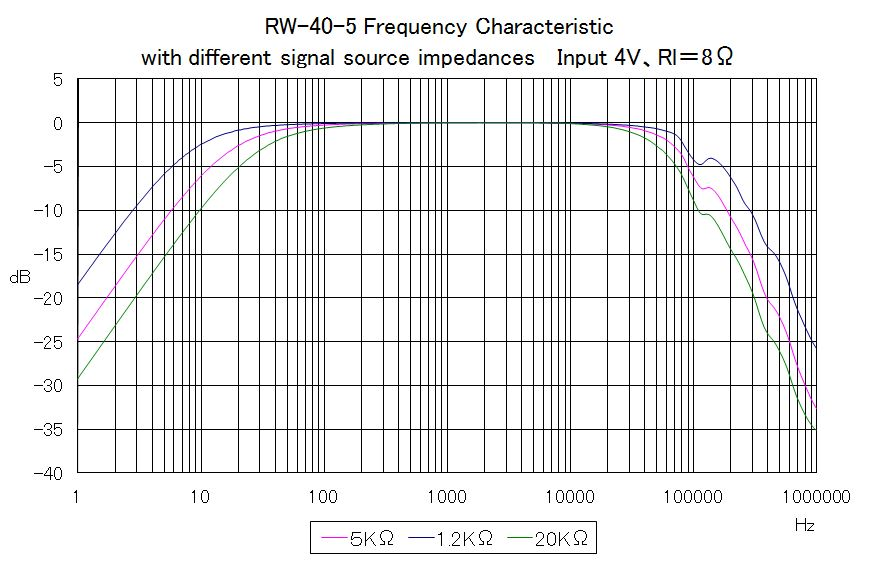Frequency bandwidth by signal source impedance

 Signal source impedance Bandwidth 5K ohms (Standard) 25Hz to 60KHz -2dB 1.2K ohms (Triode tubes) 11Hz to 80KHz -2dB 20K ohms (beam tubes) 42Hz to 44KHz -2dB

3. Phase Characteristic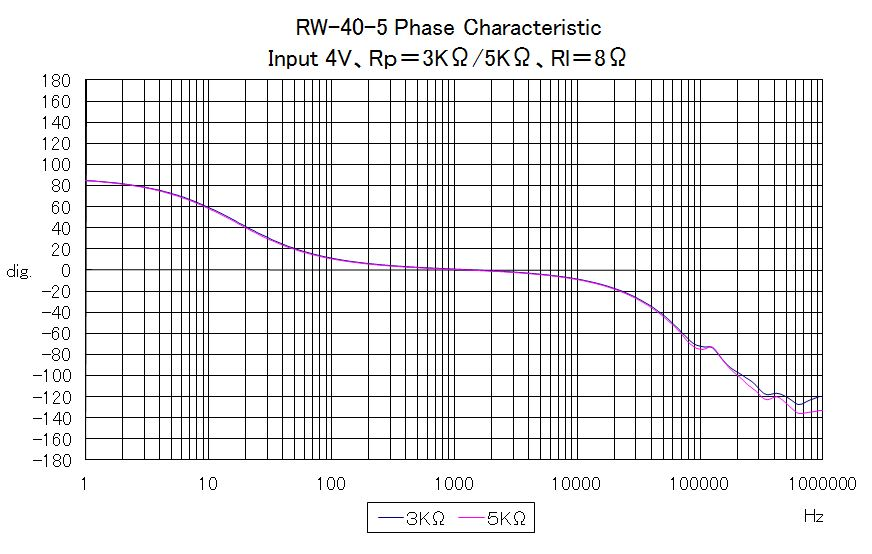4. Primary impedance Characteristic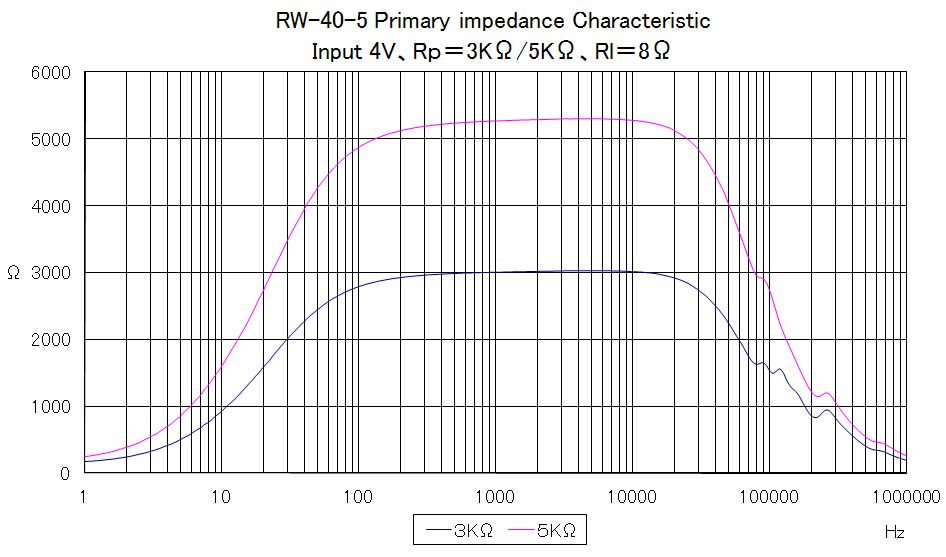5. Cathode feedback winding Frequency Characteristics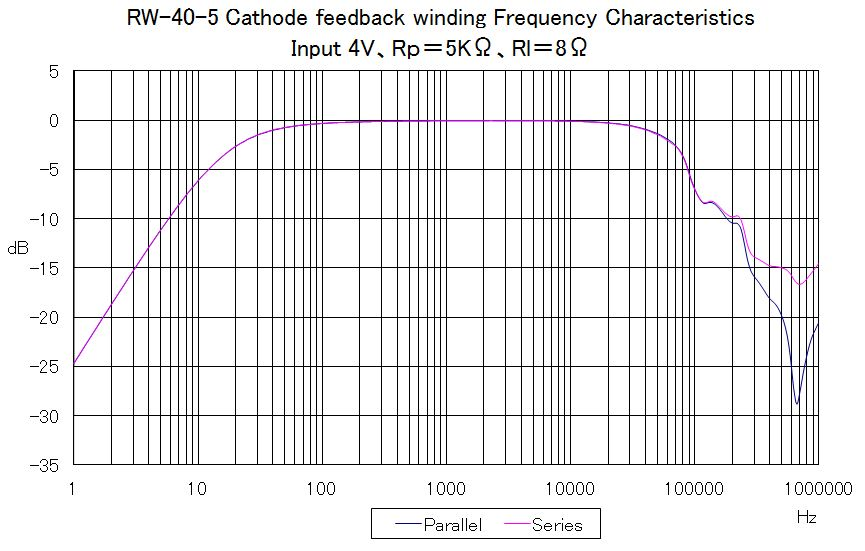6. Cathode feedback winding Phase Characteristic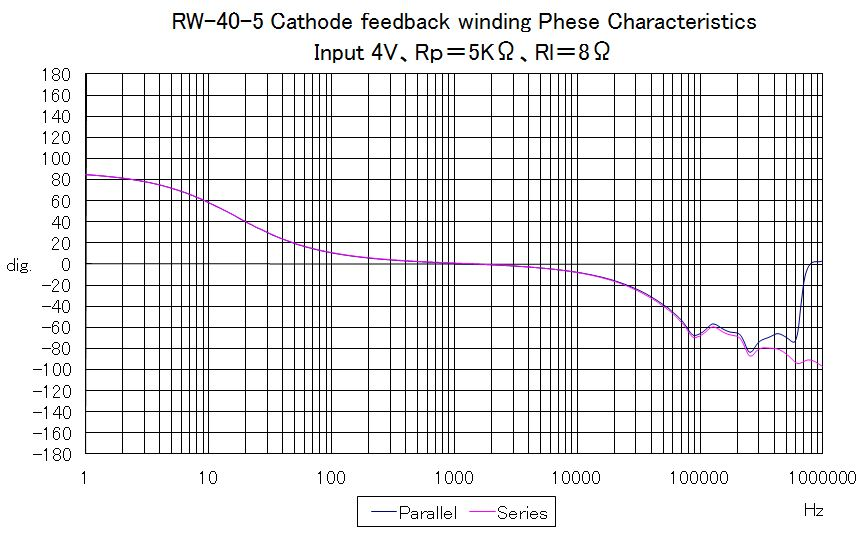7. Primary Inductance Characteristic

The primary inductance of the single-ended output transformer has a characteristic of constant inductance. With this, the output transformer can drive steadily the loudspeaker without being influenced by the level difference of the superimposed DC current or AC input signal. The less the inductance fluctuates under various conditions, the more solid and stable sound quality can be obtained.

• To make the primary inductance have higher value and less fluctuation under various conditions, well-designed core gap, generous turns of coil winding, and better magnetic properties of the core are indispensable.
• With the single-ended amplifier, superimposed DC current tends to fluctuate according to the output level change. When the primary inductance of the output transformer fluctuates because of the change of superimposed DC current, the low frequency response will also fluctuate. In other words, the low frequency response will change according to output power if the constant inductance characteristic of the output transformer is poor.
• If the core gap is widened, the constant inductance characteristic is improved, however, at the same time the value of the primary inductance decreases and consequently the low cut-off frequency will rise

Primary inductance

 Primary Minimuminductance Maximuminductance DC current 3K ohms 12H 15H 80mA 5K ohms 19H 23H 80mA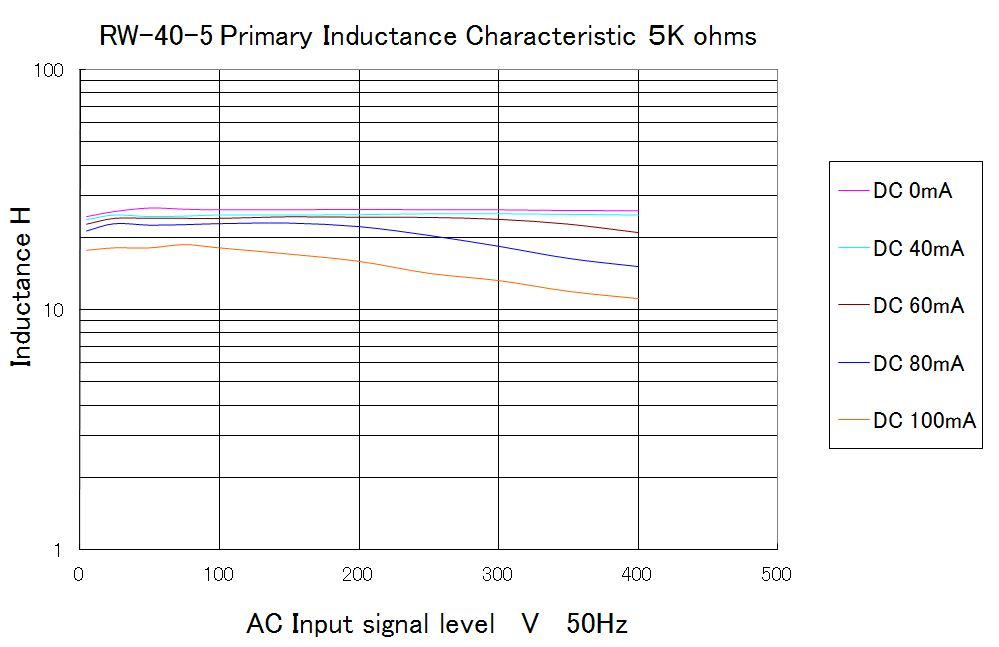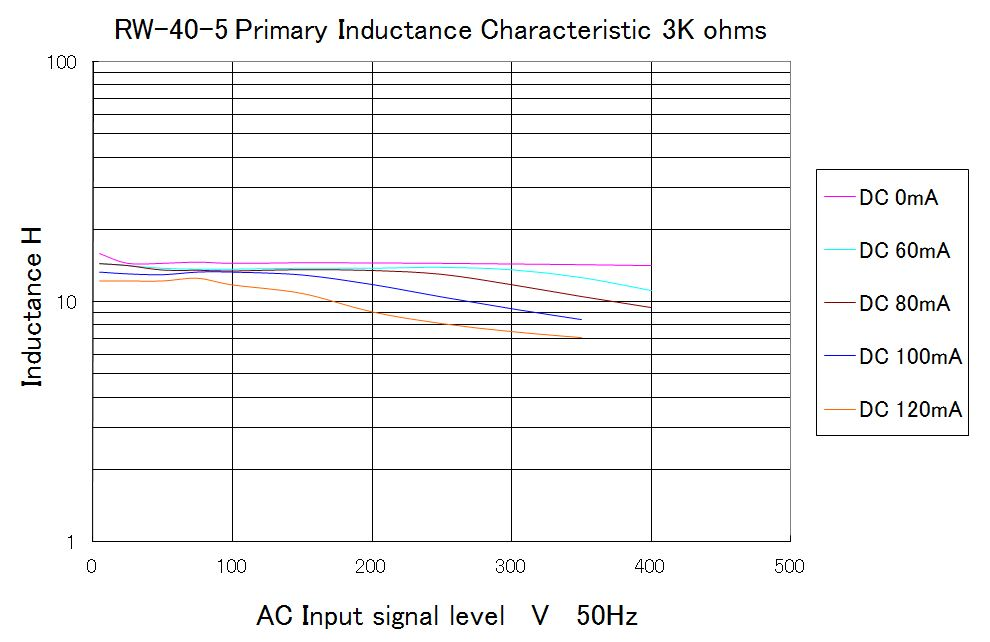### Use of RW-40-5 as push-pull output transformer

The primary coil of RW-40-5 is perfectly symmetrical the center of which is set at red lead wire. This construction enables RW-40-5 to be used as constant inductance type push-pull output transformer, fairly suitable to build a DC unbalance current proof, maintenance-free type push-pull amplifier. The table below describes the connections and specifications as constant inductance type push-pull output transformer.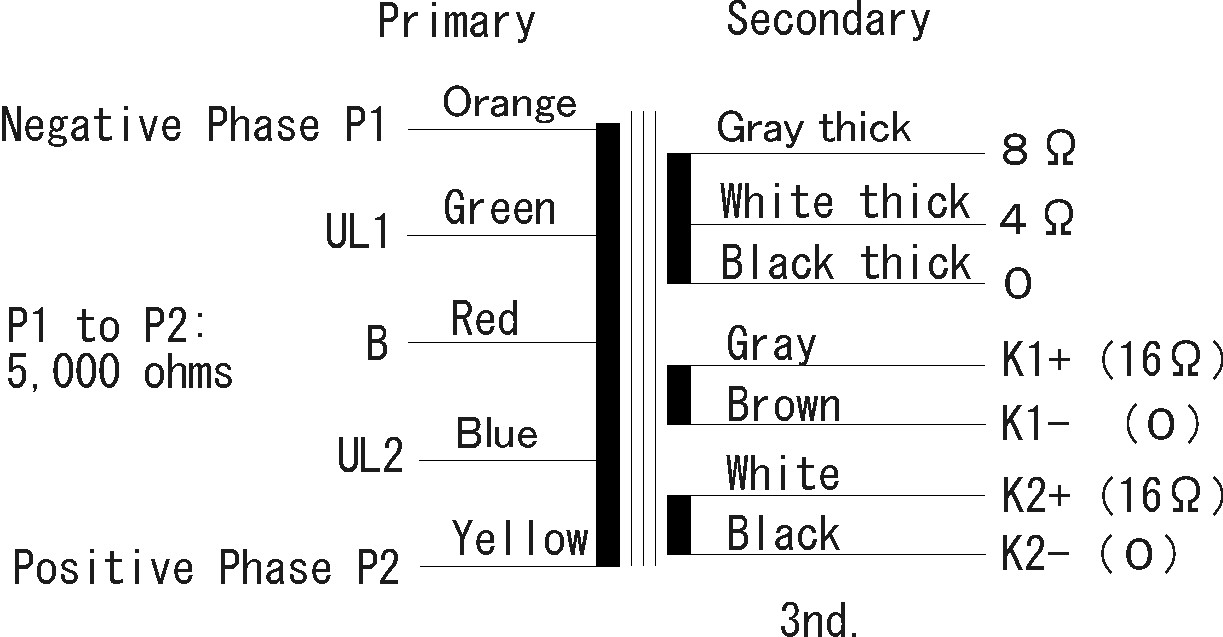Type Constant inductance type Push-Pull Output capacity 80W at 50Hz Primary impedance 5,000 ohms, with UL (50%) taps Secondary impedance 4 and 8 ohms 3rd. cathode feedback winding 16 ohms X 2 Frequency bandwidth 40Hz to 47KHz (-1dB), input=4V, signal source impedance=5,000 ohms Primary inductance (H) 19H(min.), 23H(max.) Primary permissible DC current 280mA (for 2 tubes) Primary permissible unbalance DC current 120mA (recommended within 90mA ) Power loss 0.23dB 8ohms Dielectric withstanding voltage between primary and secondary 2KV AC Maximum permissible voltage of primary P-P 1KV AC Core Type R160 160W core Shape Enclosed in square case, the same mounting dimensions as those of Tango XE60,FC-30 Connections Lead wires

1.Cathode feedback winding of push-pull use
When using the cathode feed back winding, the connection of primary winding and cathode feedback winding is as follows. In the case of self-bias, a self-bias resistance and a bypass capacitor are connected between K1-Brown and a ground and between K2+ white and a ground.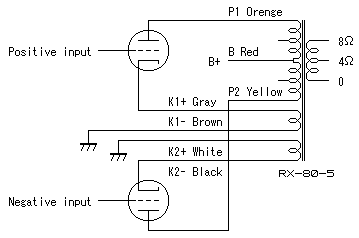Calculating the closed-loop gain and feedback ratio
The load of the output tube is both the primary winding and cathode feedback winding in the cathode feedback circuit.
The load impedance of the output tube is not 1.25K ohms of a usual pp circuit (1/4 of 5K ohms).
The load impedance is as follows.
Rl = ( SQRT(1250) + SQRT(16) ) **2 = 1548 ohms
SQRT : Square route operation
** : Factorial operation

The open-loop gain on the primary side before feedback
Aopen = gm * ( 1548 // Rp )
gm : Transconductance of the output tube
Rp : Plate resistance of the output tube
// : Parallel value operation

The feedback factor β is a voltage ratio (a winding ratio) of load Rl and the cathode winding .
β = SQRT ( 16 / 1548 ) = 0.102

The closed loop gain on the primary side after feedback
Aclosed = Aopen / ( 1 + Aopen * β )

Feedback ratio = 20 * log( Aopen / Aclosed ) dB

Example
Value that uses EL34(6CA7) for output tube
gm of EL34 = 11000uS
Rp of EL34 = 15000 ohms

The open-loop gain on the primary side before feedback
Aopen = gm * ( 1548 // Rp )
= 11000 * 10**-6 ( 1548 // 15000 )
= 11000 * 10**-6 * 1403
= 11 * 1.403 = 15.43

The closed loop gain on the primary side after feedback
Aclosed = Aopen / ( 1 + Aopen * β )
= 15.43 / ( 1 + 15.43 * 0.102 )
= 15.43 / 2.57 = 6.07
The feedback ratio
Feedback ratio = 20 * log( Aopen / Aclosed ) dB
= 20 * log( 15.43 / 6.07 ) = 8.2 dB

2. Frequency Characteristic of push-pull use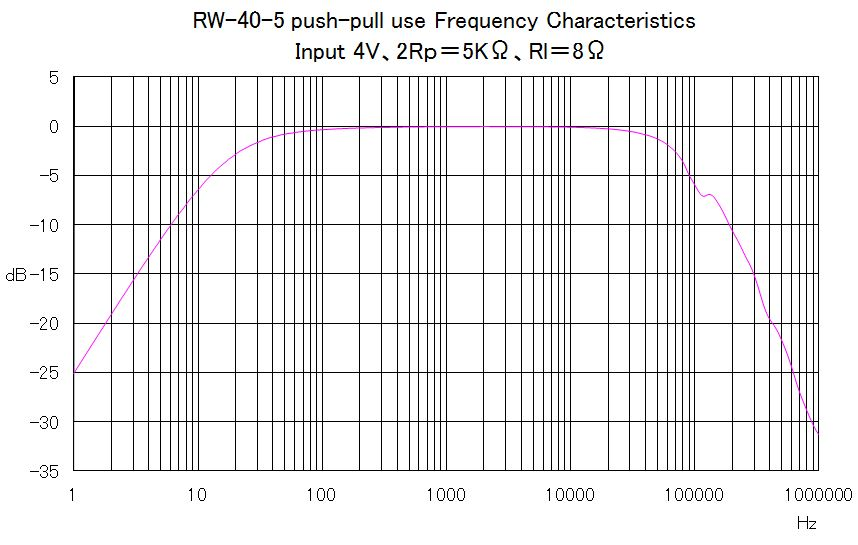3. Phase Characteristic of push-pull use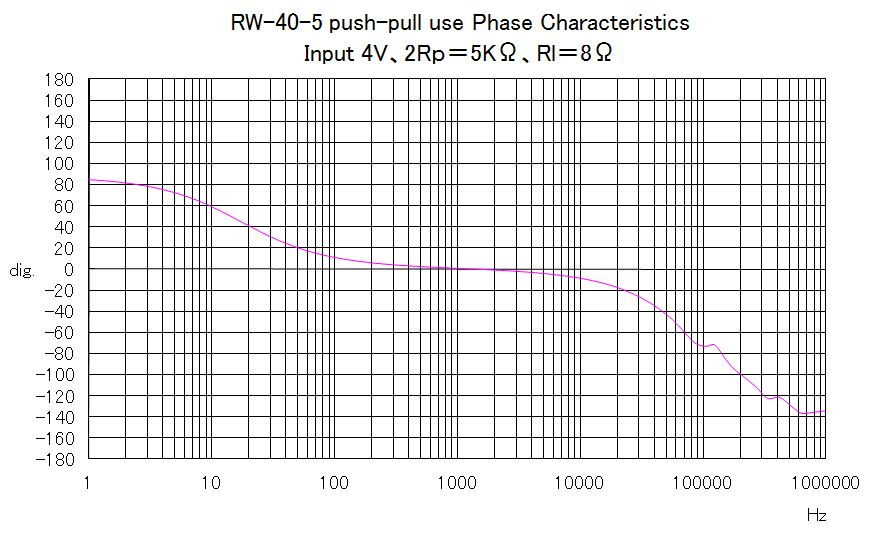4. Other Characteristics
The impedance and inductance characteristics are the same as those of the single-ended connections. Please refer to those characteristics.